# 'gggrid' it's g-g-great!Accessing 'grid' from 'ggplot2'

Version 1: Monday 31 May 2021This report describes the 'gggrid' package, which provides a convenient interface for making use of raw 'grid' functions in combination with 'ggplot2'.

The 'gggrid' package provides two functions, `grid_panel()` and `grid_group()`, both of which create a new layer in a 'ggplot2' plot. The first argument to both functions is a 'grid' grob or a function that generates a grob and that grob is added to the plot region of the 'ggplot2' plot.

For example, the following code adds a rectangle filled with a semitransparent radial gradient to a 'ggplot2' plot.

```library(gggrid)
ggplot(mtcars) +
geom_point(aes(disp, mpg)) +
```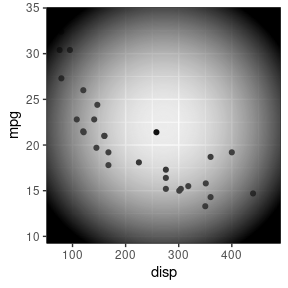## 1. Introduction

The 'grid' package for R (R Core Team, 2019) provides low-level graphics functions for arranging and drawing basic shapes. One useful feature is the ability to specify the location of drawing using a combination of coordinate systems. For example, the following code describes a text label with its top-right corner exactly 5mm in from the top-right corner of wherever it is drawn. The `y` value `unit(1, "npc") - unit(5, "mm")` is how we can say "5mm down from the top" in 'grid'.

```library(grid)
label <- textGrob("Label",
x=unit(1, "npc") - unit(5, "mm"),
y=unit(1, "npc") - unit(5, "mm"),
just=c("right", "top"))
```

In the following code, we draw a rectangle and then add the text label. This image is embellished with red lines to show the the boundary of the text and the offset of the text from the top-right corner of the image.

```grid.rect()
grid.draw(label)
```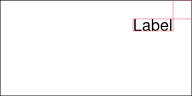The aim of this report is to explore how we can access raw 'grid' features like this in combination with data visualisations drawn by the 'ggplot2' package (Wickham, 2016).

We will spend some time in the remainder of this section establishing why there is a problem to solve, then the following section will describe a solution: The 'gggrid' package.

### The straw man:

Suppose we want to add a text label a precise distance in from the top-right corner of a 'ggplot2' plot. For example, in the plot below, the text "Label" is exactly 5mm in from the top-right corner of the plot region.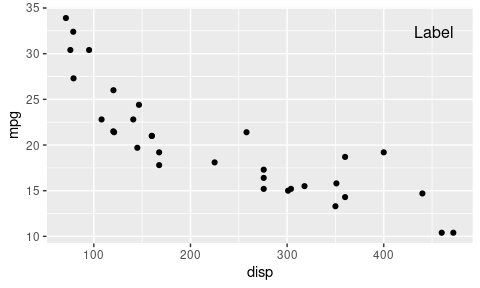This is not an easy result to produce in 'ggplot2' with standard geoms. If we use `geom_text()`, we must position the text relative to the scales on the plot. For example, we could easily place the text at the y-location 30, but calculating "5mm from the top of the plot region" in terms of the y-axis scale is not at all straightforward. Even the non-standard `annotate()` function has the same problem; the position of the text still has to be in terms of the scales on the plot.

It would be nice to be able to draw in 'ggplot2' relative to coordinate systems other than the data coordinate system within the plot region.

Yes, this could be done with `annotation_custom()`, but we will conveniently ignore that fact until later when we can explain the problems with that approach.

### The jealous man:

The 'lattice' package (Sarkar, 2008) is another package that, like 'ggplot2', uses 'grid' to draw its high-level plots. Can we produce a simple label annotation in 'lattice'?

In 'lattice', we can customise a plot by defining a "panel function", which is a function that gets called to draw the contents of the plot region. We are allowed to call any code within the panel function, including 'grid' code, so the label annotation is straightforward in 'lattice'.

In the code below, we draw a 'lattice' plot with a panel function that draws the text label we defined earlier.

```library(lattice)
xyplot(mpg ~ disp, mtcars,
panel=function(...) {
panel.xyplot(...)
grid.draw(label)
})
```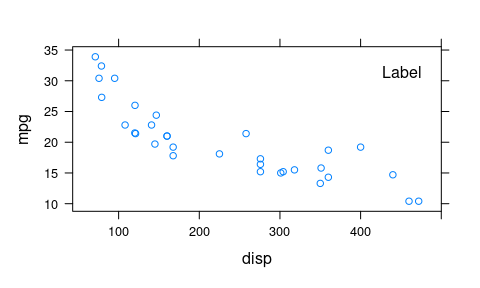It would be nice to have the equivalent of a 'lattice' "panel function" in 'ggplot2'.

One nice thing about a 'lattice' panel function is that it provides access to the useful work that 'lattice' does, including splitting the data into groups and panels and setting up useful coordinate systems. The panel function is run within the context of the plot region, which is a 'grid' viewport with the appropriate scales, and the panel function is provided with the data that is to be drawn within the plot region.

For example, the following code adds a label at a fixed position with a line to one of the data points in the 'lattice' plot. This makes use of a combination of the data values that are passed to the panel function, the coordinate system that is in place when the panel function is run, and absolute 'grid' positioning.

```library(lattice)
xyplot(mpg ~ disp, mtcars,
panel=function(x, y, ...) {
panel.xyplot(x, y, ...)
grid.text("most efficient",
x=unit(1.5, "in"),
y=unit(1, "npc") - unit(2, "lines"),
just="left")
grid.segments(unit(x[which.max(y)], "native"),
unit(max(y), "native"),
unit(1.5, "in"),
unit(1, "npc") - unit(2, "lines"),
gp=gpar(col="grey"))
})
```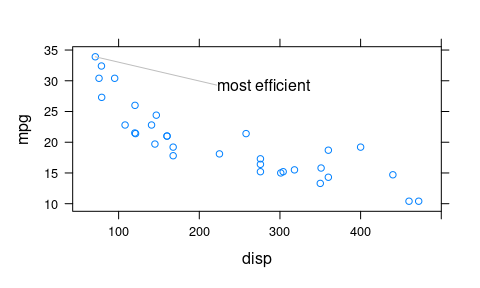It would be nice if our 'ggplot2' panel function was able to take advantage of the useful work that 'ggplot2' does, including splitting up the data and setting up useful coordinate systems.

### The heretic:

One of the reasons why 'ggplot2' is successful is because it offers a clear paradigm or philosophy for how to construct a data visualisation, based on Leland Wilkinson's Grammar of Graphics (Wilkinson, 2005). Ideas like "geoms", "aesthetics", "stats", and "coords" are central to this paradigm, but 'grid' concepts like "units" and "viewports" are not. The 'grid' concepts may also be hidden away in 'ggplot2' because they are perceived to be too awkward or complex.

However, if we are already familiar with 'grid', rigid adherence to the 'ggplot2' paradigm can sometimes mean that some things are harder or more awkward than necessary.

It would be nice to have full access from 'ggplot2' to raw 'grid', red in tooth and claw.

### The hacker:

For those intimately familiar with 'grid', there is a post-hoc way to work with a 'ggplot2' plot. The following code demonstrates the approach: having drawn a 'ggplot2' plot, we call the 'grid' function `grid.force()` to get access to all of the 'grid' grobs and viewports that 'ggplot2' created, then we can navigate to the 'grid' viewport that 'ggplot2' created for the plot region using `downViewport()`, and then we can draw the text label that we defined earlier within that context.

```ggplot(mtcars) +
geom_point(aes(disp, mpg))
grid.force()
downViewport("panel.7-5-7-5")
grid.draw(label)
```It could reasonably be argued that this approach requires deeper knowledge of 'grid' and also of 'ggplot2' than most people have or even would like to have. The `grid.force()` function and the viewport name `"panel.7-5-7-5"` are not very self-explanatory.

Furthermore, the viewport that 'ggplot2' has created does not have scales relevant to the data, so we cannot add data-based drawing. The scales on the viewport that 'ggplot2' created are just 0 to 1, not the scales that the axes show.

```current.viewport()\$yscale
```
```   0 1
```

This means that, with this post-hoc approach, although we have full access to 'grid', we cannot, for example, draw shapes relative to the plot scales, like we did in the second 'lattice' panel function example above.

### The glutton:

The 'ggplot2' package does actually allow us to specify raw 'grid' grobs in some specific cases. For example, the `annotation_custom()` function allows any 'grid' grob to be added to the plot. However, this access to 'grid' is limited. For example, the single grob that is passed to `annotation_custom()` is drawn in every panel of a facetted plot, it is positioned within a region that is defined in terms of the plot scales, and it has no access to the aesthetic mappings for the plot.

The following code shows how our simple label could be added using `annotation_custom()`.

```ggplot(mtcars) +
geom_point(aes(disp, mpg)) +
annotation_custom(label)
```That is simple enough, but what if we use facetting? All we can get is the same grob (in the same place) in every panel.

```ggplot(mtcars) +
geom_point(aes(disp, mpg)) +
facet_wrap("manual") +
annotation_custom(label)
```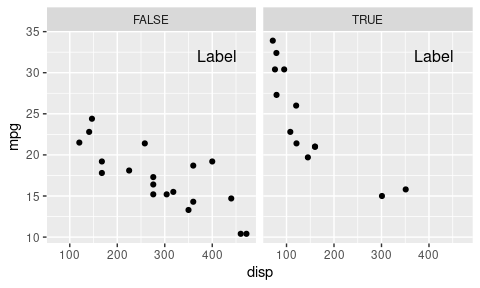Furthermore, as with the post-hoc approach, we do not have access to the 'ggplot2' coordinate system, so we cannot draw a grob relative to the axis scales.

Did I mention that it would be nice to have access to 'grid' and access to the 'ggplot2' context at the same time? I want it all!

There are two functions that I know of that provide a variation on `ggplot2::annotation_custom()`: `egg::geom_custom()` and `ggpmisc::geom_grob()`. The reasons why these are still not what I want are left to the Discussion.

Although 'ggplot2' uses basic 'grid' shapes to draw its Geoms, there are some 'grid' shapes, or 'grid'-based packages, that are not accessible from 'ggplot2'. For example, the 'vwline' package (Murrell, 2019b) draws variable-width lines and the 'gridGeometry' package (Murrell, 2019a) provides constructive geometry operations on 'grid' grobs.

Users are currently dependent on a developer creating a Geom interface in order to use the full range of 'grid'-based shapes in a 'ggplot2' plot.

It would be nice to have instant access to ALL 'grid' shapes within a 'ggplot2' plot.

I did find some mentions online of a github package 'ggvwline' by Houyun Huang, but the links were all stale.

## 2. The 'gggrid' package

The idea behind the 'gggrid' package is to allow the user to compose a data visualisation from a combination of 'ggplot2' output and 'grid' output. The user should be able to make use of the advantages of 'ggplot2' where that makes sense, e.g., to describe the essential structure of a complex image at a high level, and at the same time make use of the advantages of 'grid' where that makes sense, e.g., to specify precise locations relative to a range of coordinate systems.

There are two functions in the 'gggrid' package: `grid_panel()` and `grid_group()`. Both functions add a new layer to a 'ggplot2' plot, but they deliberately do not follow the typical naming scheme of `geom_*` or `stat_*` because these functions are not trying to strictly adhere to the 'ggplot2' paradigm; they add raw 'grid' drawing to a 'ggplot2' plot.

In the simplest case, we call `grid_panel()` with a 'grid' grob as the only argument. For example, the following code produces our precisely positioned label from the very beginning of the report.

```ggplot(mtcars, aes(disp, mpg)) +
geom_point() +
grid_panel(label)
```The 'grid' grob can be more complex than a simple shape. For example, the following code adds a gTree that draws a combination of a rectangle and a text label, both within a new 'grid' viewport that is pushed within the current 'ggplot2' plot region.

```bg <- rectGrob(gp=gpar(fill="black"))
lab <- textGrob("mtcars", gp=gpar(col="white"))
vp <- viewport(x=1, y=1, width=.5, height=unit(1, "lines"),
just=c("right", "top"))
banner <- gTree(children=gList(bg, lab), vp=vp)
ggplot(mtcars, aes(disp, mpg)) +
geom_point() +
grid_panel(banner)
```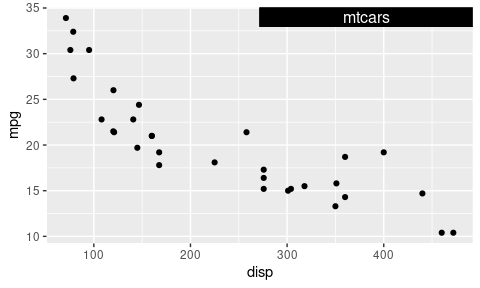It is important to note that we already have some access to the 'ggplot2' context, even when we only provide a fixed grob to `grid_panel`; the grob is drawn within the 'grid' viewport that represents the 'ggplot2' plot region. For example, the following code simply adds an empty 'grid' rectangle (with a thick border). This is by default the same size as the viewport it is drawn within, so we see a rectangle around the 'ggplot2' plot region.

```rect <- rectGrob(gp=gpar(fill=NA, lwd=10))
ggplot(mtcars, aes(disp, mpg)) +
geom_point() +
grid_panel(rect)
```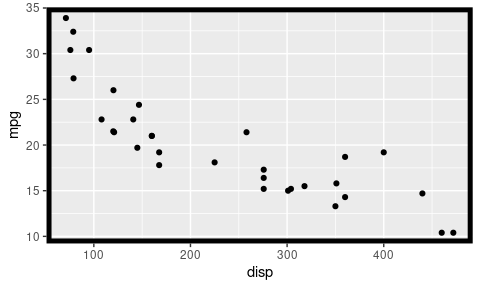As we saw earlier, the scales on the 'grid' viewport that 'ggplot2' creates for the plot region do not reflect the axis scales, so when we just call `grid_panel()` with a grob as the first argument, we do not have full access to the 'ggplot2' context for the plot region. However, if we provide a function as the first argument to `grid_panel()`, that function is passed the `data` and the `coords` (the transformed data) for the plot, which provides us with enough information to start writing "panel functions" in the sense of the 'lattice' package.

As a simple example, the following code calls `grid_panel()` with a function that generates a 'grid' grob based on the largest and smallest data values. The `coords` are values that have already been transformed to the plot scales, so we can just draw a 'grid' rectangle around the minimum and maximum of those values.

```rectFun <- function(data, coords) {
left <- min(coords\$x)
bottom <- min(coords\$y)
width <- diff(range(coords\$x))
height <- diff(range(coords\$y))
rectGrob(left, bottom, width, height,
just=c("left", "bottom"),
gp=gpar(fill=NA, lwd=1))
}
ggplot(mtcars, aes(x=disp, y=mpg)) +
geom_point() +
grid_panel(rectFun)
```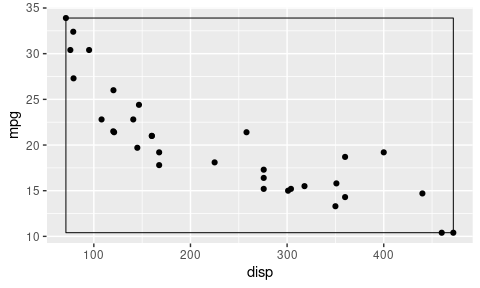It is important to note that the columns names for the `data` and `coords` that are passed to `rectFun()` in the above example come from the aesthetic mappings in the 'ggplot2' plot. For example, in this case, we have mapped the `disp` column of the `mtcars` data set to the `x` aesthetic, so both `data` and `coords` have a column named `x`. Both `grid_panel()` and `grid_group()` provide a `debug` argument that can be a function and this can be used to inspect the values that are being passed to the `grob` function.

```debugHead <- function(data, coords) {
}
ggplot(mtcars, aes(x=disp, y=mpg)) +
geom_point() +
```
```      x    y PANEL group
1 160 21.0     1    -1
2 160 21.0     1    -1
3 108 22.8     1    -1
4 258 21.4     1    -1
5 360 18.7     1    -1
6 225 18.1     1    -1
x         y PANEL group
1 0.2470464 0.4555126     1    -1
2 0.2470464 0.4555126     1    -1
3 0.1291299 0.5251451     1    -1
4 0.4692737 0.4709865     1    -1
5 0.7005714 0.3665377     1    -1
6 0.3944421 0.3433269     1    -1
```

The following code provides a slightly more complex example that demonstrates the combination of 'ggplot2' context (the data values) and raw 'grid'. In this case, we are drawing a "rug" of short lines at the right edge of the plot. On one hand, the y-location of the lines are based on the 'ggplot2' data, but on the other hand, the length of the lines (2mm) is specified using 'grid' units.

Yes, there is a `geom_rug()`, but this is a nice practical and easy-to-understand example to start with. Later examples will go to some places that 'ggplot2' cannot currently go.

```rug <- function(data, coords) {
segmentsGrob(unit(1, "npc"),
coords\$y,
unit(1, "npc") - unit(2, "mm"),
coords\$y,
gp=gpar(lwd=2, col=rgb(0,0,0,.5)))
}
ggplot(mtcars, aes(x=disp, y=mpg)) +
geom_point() +
grid_panel(rug)
```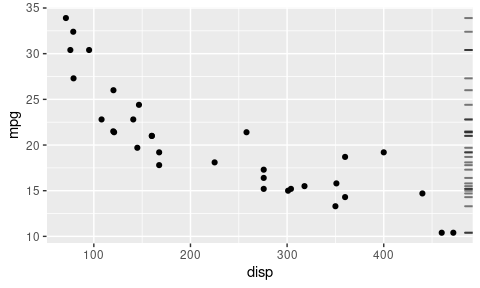Another advantage of specifying a `grob` function is that it is evaluated for each panel. The following code demonstrates this by adding facetting (but just reusing the `rug()` function); we get a (different) rug added to each panel.

```ggplot(mtcars, aes(x=disp, y=mpg)) +
geom_point() +
facet_wrap("manual") +
grid_panel(rug)
```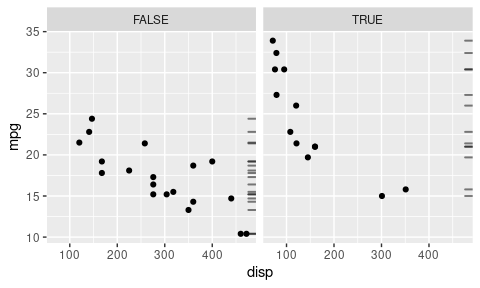This rule also holds for the `grid_group()` function. If there are distinct groups being drawn in the plot, `grid_group()` will call the `grob` function for each group. For example, the following code produces a 'ggplot2' plot with two groups of points differentiated by `colour`. We call `grid_group()` with a new "rug" grob function that colours the short lines for each group based on the colour used for the data.

```rugGroup <- function(data, coords) {
segmentsGrob(unit(1, "npc"),
coords\$y,
unit(1, "npc") - unit(2, "mm"),
coords\$y,
}
ggplot(mtcars, aes(x=disp, y=mpg, color=manual)) +
geom_point() +
grid_group(rugGroup)
```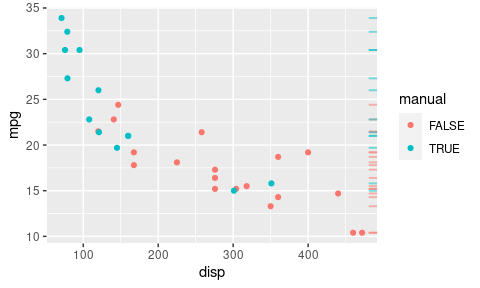In addition to data values and transformed data values, the `grid_panel()` and `grid_group()` functions have access to any variables that are calculated by 'ggplot2' "stats". For example, the following code shows the variables that are available if we use `stat_smooth`.

```ggplot(mtcars, aes(x=disp, y=mpg, color=manual)) +
geom_point() +
```
```     colour        x        y     ymin     ymax       se flipped_aes PANEL group
1 #F8766D 120.1000 22.62935 18.88379 26.37491 1.745393       FALSE     1     1
2 #F8766D 124.5544 22.40251 19.04756 25.75746 1.563373       FALSE     1     1
3 #F8766D 129.0089 22.17798 19.16829 25.18768 1.402487       FALSE     1     1
4 #F8766D 133.4633 21.95860 19.24232 24.67488 1.265760       FALSE     1     1
5 #F8766D 137.9177 21.74720 19.26744 24.22696 1.155544       FALSE     1     1
6 #F8766D 142.3722 21.54623 19.24401 23.84846 1.072815       FALSE     1     1
colour         x         y      ymin      ymax       se flipped_aes PANEL group
1 #F8766D 0.1565682 0.5139397 0.4001499 0.6277295 1.745393       FALSE     1     1
2 #F8766D 0.1666692 0.5070483 0.4051252 0.6089715 1.563373       FALSE     1     1
3 #F8766D 0.1767701 0.5002273 0.4087930 0.5916616 1.402487       FALSE     1     1
4 #F8766D 0.1868711 0.4935625 0.4110421 0.5760829 1.265760       FALSE     1     1
5 #F8766D 0.1969721 0.4871401 0.4118051 0.5624751 1.155544       FALSE     1     1
6 #F8766D 0.2070731 0.4810347 0.4110932 0.5509762 1.072815       FALSE     1     1
```

This allows us to add 'grid' drawing based on "stat" output, as shown below. In this case, we add a label parallel to the smooth line and we calculate the angle of the text using the `x` and `y` values that come from the "stat" smooth.

```addLabel <- function(data, coords) {
label <- expression(y == alpha + beta*x + epsilon)
xpos <- which(coords\$x > .5)
dx <- convertWidth(unit(diff(coords\$x[1:2]), "npc"), "in", valueOnly=TRUE)
dy <- convertHeight(unit(diff(coords\$y[1:2]), "npc"), "in", valueOnly=TRUE)
angle <- 180*atan2(dy, dx)/pi
textGrob(label,
x=unit(coords\$x[xpos], "npc"),
y=unit(coords\$y[xpos], "npc") + unit(.5, "mm"),
just="bottom",
rot=angle,
gp=gpar(col=2))
}
ggplot(mtcars, aes(x=disp, y=mpg)) +
geom_point() +
geom_smooth(method="lm", colour=2) +
```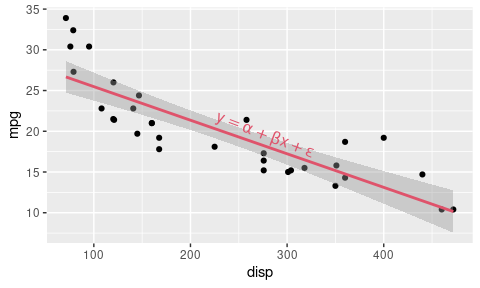We can also use aesthetic mappings to pass additional information to `grid_panel`. For example, in the following code we make sure that the vehicle names are included in the `data` that are passed to the grob-generating function, `nameVehicle()`, by specifying `aes(label=name)`. The `nameVehicle()` function uses this information, along with the default `data` and `coords` values, to label the most efficient vehicle. We use facetting to emphasise that the calculations occur for each panel.

This example also highlights the fact that 'gggrid' is not playing by all of the 'ggplot2' rules because it generates a warning about unknown aesthetics.

```nameVehicle <- function(data, coords) {
text <- textGrob(data\$label[which.max(coords\$y)],
x=unit(1, "npc") - unit(5, "mm"),
y=unit(1, "npc") - unit(2, "lines"),
just="right")
line <- segmentsGrob(coords\$x[which.max(coords\$y)],
max(coords\$y),
unit(1, "npc") - unit(5, "mm") -
grobWidth(text),
unit(1, "npc") - unit(2, "lines"),
gp=gpar(col="grey"))
gTree(children=gList(line, text))
}
ggplot(mtcars, aes(disp, mpg)) +
geom_point() +
facet_wrap("manual") +
grid_panel(nameVehicle, aes(label=name))
```
```  Warning: Ignoring unknown aesthetics: label
```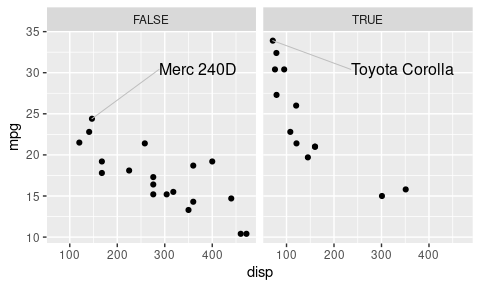The next example demonstrates the idea of accessing 'grid'-based drawing that does not (yet) have a 'ggplot2' Geom interface. The following code makes use of the 'vwline' package to draw a variation on Minard's famous map. The `grid_group()` function is useful here because there is no existing 'ggplot2' Geom interface to the 'vwline' package, so we need direct access to the raw 'grid'-based function `vwlineGrob()`.

This example is also interesting because there are no `geom_*()` calls; `grid_panel()` is the only layer in the plot. Furthermore, we make good use of the 'ggplot2' infrastructure to set up the coordinate system for the plot, using `coord_fixed()`, and the mapping from the number of survivors to the line width, using `scale_size()`. This makes our grob-generating function, `path()`, quite straightforward.

The data for this example comes from the supplementary materials published with Wickham, 2010.

```minard <- read.table("Minard/minard-troops.txt", header=TRUE)
library(vwline)
path <- function(data, coords) {
vwlineGrob(coords\$x, coords\$y, w=unit(coords\$size, "in"),
gp=gpar(col=coords\$colour,
}
ggplot(minard,
aes(x=long, y=lat, size=survivors, colour=direction,
group=interaction(group, direction))) +
scale_size(range=c(.01, .5)) +
coord_fixed(2, clip="off") +
grid_group(path)
```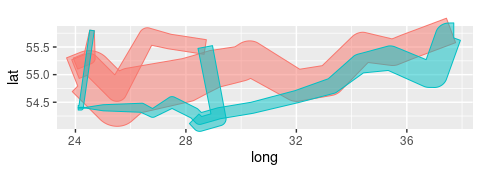The final example demonstrates a combination of 'gggrid' and post-hoc editing of 'ggplot2' plots. This example makes use of a lot of raw 'grid' tools and techniques, so requires a little more explanation.

First, as part of the main 'ggplot2' plot, we call `grid_panel()` just to add a "null" grob at the location of the data symbol representing the highest `mpg` (in each panel), but we do not immediately draw the 'ggplot2' plot. Instead, we push a 'grid' viewport that leaves a gap at the top of the page and draw the 'ggplot2' plot in the remainder of the page. We define a text grob and draw that in the gap at the top of the page. We then call `grid.force()` to make the 'grid' grobs and viewports from the 'ggplot2' plot accessible and we determine the "path"s to the "null" grob markers that we drew on the 'ggplot2' plot (and to the viewports that those markers were drawn within). For each marker, we navigate down to the viewport that the marker was drawn within, calculate the location of the marker in terms of the entire page, navigate back up to the "root" viewport (the whole page), and draw a curved line (with an arrow) from the right edge of the text label to the marker.

```marker <- function(data, coords) {
nullGrob(coords\$x[which.max(coords\$y)],
max(coords\$y),
name="mark")
}
g <- ggplot(mtcars, aes(disp, mpg)) +
geom_point() +
facet_wrap("manual") +
grid_panel(marker) +
coord_cartesian(clip="off")
grid.newpage()
pushViewport(viewport(y=0,
height=unit(1, "npc") - unit(2, "lines"),
just="bottom",
name="ggplot"))
plot(g, newpage=FALSE)
upViewport()
text <- textGrob("most efficient ",
x=unit(1, "lines"),
y=unit(1, "npc") - unit(1, "lines"),
just=c("left", "top"))
grid.draw(text)
grid.force()
markers <- grid.grep("mark", global=TRUE, viewports=TRUE)
drawLine <- function(x) {
depth <- downViewport(attr(x, "vpPath"))
m <- grid.get(x)
loc <- deviceLoc(m\$x, m\$y)
upViewport(depth)
grid.curve(grobX(text, 0), grobY(text, 0),
loc\$x, loc\$y,
square=FALSE, curvature=-.5, angle=45, ncp=4,
arrow=arrow(length=unit(3, "mm"), angle=20, type="closed"),
gp=gpar(col="grey", fill="grey"))
}
lapply(markers, drawLine)
```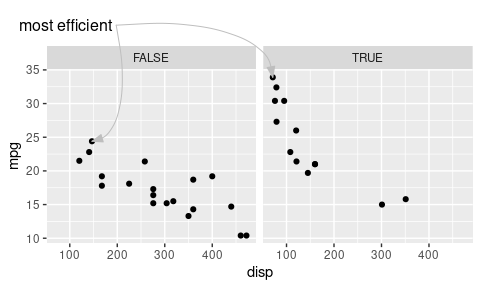## 3. Discussion

There is not a lot of code in the 'gggrid' package. The main contribution of the package is possibly just a change in mindset, to embrace the use of raw 'grid' in combination with 'ggplot2', rather than trying to avoid raw 'grid' as much as possible.

What the 'gggrid' package provides is full access to ALL 'grid' features, including units and viewports, gradient and pattern fills, and 'grid'-based drawing such as variable-width lines and constructive geometry.

### Custom Geoms

Anyone who has developed a custom 'ggplot2' Geom may have recognised that all of the examples could have been achieved by creating a special Geom every time instead of using `grid_panel()` or `grid_group()`. Nevertheless, 'gggrid' saves on quite a bit of typing. In effect, 'gggrid' allows us to develop a new 'ggplot2' Geom on-the-fly (while flouting some of the normal rules, like having to formally declare the aesthetics that our Geom supports). From this perspective, 'gggrid' may provide a useful intermediary between naive 'ggplot2' user and hard-core 'ggplot2' Geom developer.

### Related work

There are two packages with functions that allow raw 'grid' grobs to be added to 'ggplot2' plots: The `geom_custom()` function from the 'egg' package (Auguie, 2019) and `geom_grob()` from the 'ggpmisc' package (Aphalo, 2021).

The `geom_custom()` function allows the user to provide a `grob_fun` argument, which is a function that generates a grob, similar to providing a function to `grid_panel()`. However, with `geom_custom()`, that `grob_fun` function is called once for each row of the data set being plotted (and the `x` and `y` components of the resulting grob are then set based on the data set). Furthermore, `geom_custom()` requires a `data` aesthetic (i.e., a `data` column within the data set), that provides the data values that are sent to the `grob_fun`. This interface is designed specifically for adding a grob for each row of the data set and it is both awkward for simpler tasks, such as adding a single label, and restrictive for more complex tasks, such as adding a single label using calculations based on the entire data set.

The `geom_grob()` function from the 'ggpmisc' package requires the user to provide a column of 'grid' grobs in the data set. Each of these grobs is then drawn within a viewport that is based on the `x` and `y` aesthetics in the data set. Again, the design is aimed at drawing a grob for each row of the data set and again it makes simpler tasks awkward and more complex tasks quite difficult.

In both cases, the functions that allow 'grid' grobs to be added to a 'ggplot2' plot appear to be constrained by their conformance to the 'ggplot2' philosophy. The 'gggrid' package deliberately ignores parts of the standard 'ggplot2' approach in order to provide unfettered access to 'grid'.

## 4. Summary

The philosophy of the 'ggplot2' package has no room for some important 'grid' concepts, like units and viewports. This means that some things are harder to do than they need to be. The 'gggrid' package offers the opportunity to break with the orthodoxy in order to make some things easier to draw.

The goal of 'gggrid' is both to make it easy to perform simple 'grid' drawing and to make it possible to perform more complex 'grid' drawing, with full access to both 'grid' and the 'ggplot2' context. Simple tasks are made easy by providing a single grob as the first argument to `grid_panel()`, in which case the 'ggplot2' data are entirely ignored, though drawing still occurs in the 'ggplot2' plot region. Complex tasks are made possible by providing a function as the first argument to `grid_panel`, in which case the 'ggplot2' data are available to base drawing on, as well as all 'ggplot2' aesthetic mappings, calculated values from "stats", and scale and coordinate transformations.

## 5. Technical requirements

The examples and discussion in this document relate to version 0.1-0 of the 'gggrid' package. Some examples also require R version 4.1.0 or later.

This report was generated within a Docker container (see the Resources section below).

## 6. Resources

• The raw source file for this report, a valid XML transformation of the source file, a 'knitr' document generated from the XML file, two R files and the bibtex file that are used to generate the table of contents and reference sections, two XSL files and an R file that are used to transform the XML to the 'knitr' document, and a Makefile that contains code for the other transformations and coordinates everything. These materials are also available on github.
• This report was generated within a Docker container. The Docker command to build the report is included in the Makefile above. The Docker image for the container is available from Docker Hub; alternatively, the image can be rebuilt from its Dockerfile.

## How to cite this document

Murrell, P. (2021). "Accessing 'grid' from 'ggplot2'" Technical Report 2021-01, Department of Statistics, The University of Auckland. version 1. [ bib | DOI | http ]

## 7. References

[Aphalo, 2021]
Aphalo, P. J. (2021). ggpmisc: Miscellaneous Extensions to 'ggplot2'. R package version 0.3.9. [ bib | http ]
[Auguie, 2019]
Auguie, B. (2019). egg: Extensions for 'ggplot2': Custom Geom, Custom Themes, Plot Alignment, Labelled Panels, Symmetric Scales, and Fixed Panel Size. R package version 0.4.5. [ bib | http ]
[Murrell, 2019a]
Murrell, P. (2019a). gridGeometry: Polygon Geometry in 'grid'. R package version 0.2-0. [ bib | http ]
[Murrell, 2019b]
Murrell, P. (2019b). vwline: Draw Variable-Width Lines. R package version 0.2-2. [ bib | http ]
[R Core Team, 2019]
R Core Team (2019). R: A Language and Environment for Statistical Computing. R Foundation for Statistical Computing, Vienna, Austria. [ bib | http ]
[Sarkar, 2008]
Sarkar, D. (2008). Lattice: Multivariate Data Visualization with R. Springer, New York. ISBN 978-0-387-75968-5. [ bib | http ]
[Wickham, 2010]
Wickham, H. (2010). A layered grammar of graphics. Journal of Computational and Graphical Statistics, 19(1):3--28. [ bib | DOI | arXiv | www: ]
[Wickham, 2016]
Wickham, H. (2016). ggplot2: Elegant Graphics for Data Analysis. Springer-Verlag New York. [ bib | http ]
[Wilkinson, 2005]
Wilkinson, L. (2005). The Grammar of Graphics (Statistics and Computing). Springer-Verlag, Berlin, Heidelberg. [ bib ]